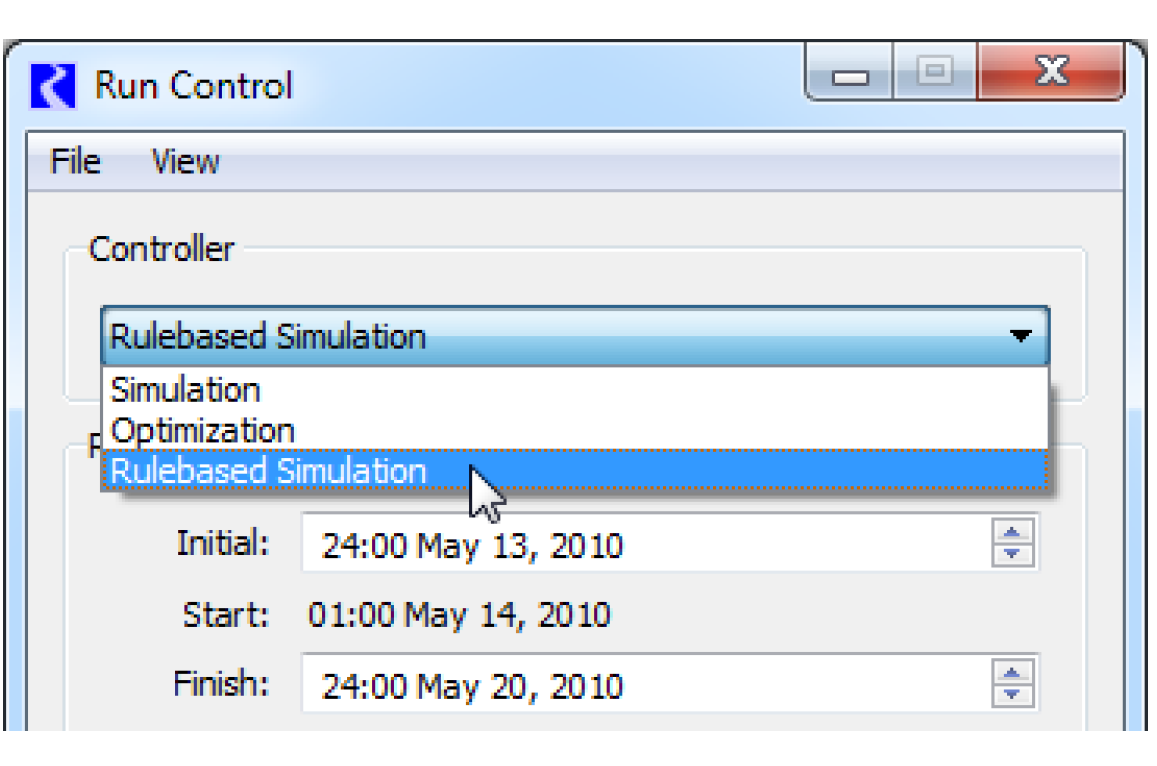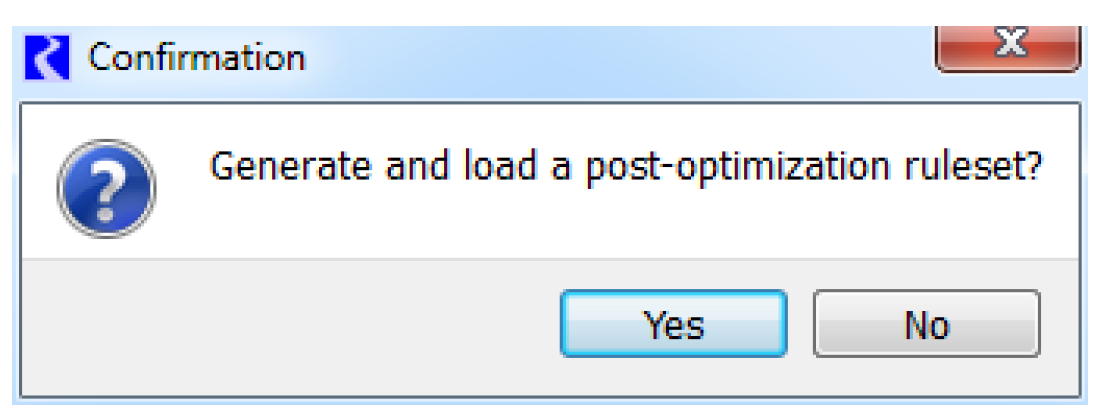Optimization : Combining Optimization With Simulation : Standard Controller Sequence
Standard Controller Sequence
A standard RiverWare Optimization run uses the Simulation - Optimization - Rulebased Simulation controller approach as follows: a Simulation run is made to clear any output values, check data, and propagate input values, then the Optimization is performed, and finally, a Post-optimization Rulebased Simulation is run to set the optimal values on the objects. Every run should be made as follows:
• Switch the run controller to Simulation, and select Start. Let the model run to completion.
• Switch the run controller to Optimization, load a Goal Set (if not already loaded), and select Start. Let the model run to completion.
• Switch the run controller to Rulebased Simulation, load a Ruleset (if not already loaded), and select Start. Let the model run to completion.
The use of the three controllers is described in more detail in the following sections.
Simulation
The user starts with a model set up to run with the Simulation controller. The Simulation run serves five purposes described below.
Clear Output Values
This guarantees that leftover output data from a previous solution does not inadvertently get used in the current run.
Check for Required Inputs
Many Simulation methods check for required input data at the start of the Simulation run.
Preprocessing of Data
If the model contains initialization rules or expression slots that evaluate at the beginning of the run to preprocess data, these get evaluated during the simulation.
Propagate Input Values
Input values on linked slots get propagated across the links in the Simulation run so that they are properly registered as values for the corresponding variables.
Simulate where Possible
The Simulation run can also be used to simulate all aspects of the basin for which the user does not want to optimize. For example, the user might know that one reservoir may only release minimum flows during the run. Then the Outflow for that reservoir can be set as input to the minimum flow value for all timesteps, and the Simulation run will propagate the effect of this. Then in the optimization run, this reservoir is not considered.
Note:  A minimum flow requirement could alternatively be set by a high priority constraint in the Optimization Goal Set.
In most cases, after the Simulation run most slots of interest will still display NaN.
Optimization
The solution of the linear goal program takes place during the Optimization run. During this run RiverWare takes the combination of the prioritized Optimization Goal Set and the model data (topology, physical characteristic data, time series data) and uses them to formulate a series of linear programs which it sends to CPLEX. CPLEX solves each linear program and returns the optimal values of the variables to RiverWare. See Mathematics of Preemptive Linear Goal Programming for details of the preemptive linear goal programming solution.
At the end of the Optimization run, RiverWare stores the final optimal value for every variable. At this point, no values have been set in slots on the workspace. That is to say that all slots which displayed NaN after the Simulation run will still display NaN after the Optimization run. Values will not be set in slots until the Post-optimization Rulebased Simulation, which is described in the following section.
Post-optimization Rulebased Simulation
After the run with the Optimization controller is completed, the Optimization solution is stored internally in RiverWare. Values from the solution are not yet returned to the workspace. All slots that displayed NaN at the end of the Simulation run will continue to display NaN at the end of the Optimization run. The solution is returned to the workspace by running a Post-optimization Rulebased Simulation. This is a special use of the RiverWare Rulebased Simulation (RBS) controller. The Ruleset for a Post-optimization RBS does not typically reflect the operating policy of the basin as it would for a standard RBS. The operating policy is expressed in the Optimization Goal Set (though there is technically nothing to prevent formulating rules in the Post-optimization ruleset to override results from the Optimization solution with additional policies). Rather the rules in a Post-optimization ruleset set select values on the simulation objects, typically reservoir outflows, based on the values from the Optimization solution. With these values set, the simulation can solve fully for all remaining variables.
A second purpose of the Post-optimization RBS is to remove approximation error introduced by linear approximations in the Optimization solution. For example, Power must be linearized in the Optimization solution, introducing approximation error into the solution for Power. In the Post-optimization RBS, the reservoirs solve for Power using the full non-linear simulation method given the Outflow specified by the Optimization solution. This removes the approximation error introduced into Power from the linearization employed for Optimization.
After the Optimization run is complete, switch the Controller to Rulebased Simulation.If no Ruleset is loaded when the controller is changed from Optimization to Rulebased Simulation, a message will appear asking if the user wants RiverWare to generate and load a ruleset. Either select Yes to use this automatically generated Ruleset for the Post-optimization RBS, or create your own custom set. See Post-optimization Ruleset for descriptions of these two options.Revised: 08/02/2021